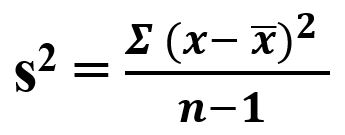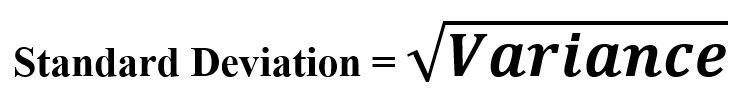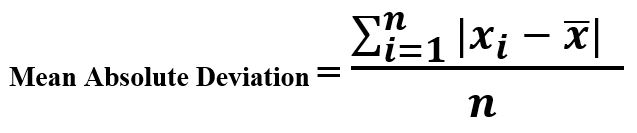# Estimation of Variable | set 1

Variability: It is the import dimension which measures the data variation i.e. whether the data is spread out or tightly clustered. Also known as Dispersion When working on data sets in Machine Learning or Data Science, it involves many steps – variance measurement, reduction, distinguishing random variability from the real one. identifying sources of real variability, making decisions regarding the pre-processing choice or model selection based on it.

Terms related to Variability Metrics :

```-> Deviation
-> Variance
-> Standard Deviation
-> Mean Absolute Deviation
-> Order Statistics
-> Range
-> Percentile
-> Inter-quartile Range
```
• Deviation : We can call it – errors or residuals also. It is the measure of how different/dispersed the values are, from the central/observed value.
Example :

```Sequence : [2, 3, 5, 6, 7, 9]
Suppose, Central/Observed Value = 7

Deviation = [-5, -4, -2, -1, 0, 2]
```
• Variance (s2): It is the best known measure to estimate the variablilty as it is Squared Deviation. One can call it mean squared error as it is the average of standard deviaiton.Example :

```Sequence : [2, 3, 5, 6, 7, 9]
Mean              = 5.33
Total Terms, n    = 6
Squared Deviation = (2 - 5.33)2 + (3 - 5.33)2 + (5 - 5.33)2
(6 - 5.33)2 + (7 - 5.33)2 + (9 - 5.33)2
Variance          = Squared Deviation / n
```

Code –

 `# Variance ` ` `  `import` `numpy as np ` ` `  `Sequence ``=` `[``2``, ``3``, ``5``, ``6``, ``7``, ``9``] ` ` `  `var ``=` `np.var(Sequence) ` ` `  `print``(``"Variance : "``, var) `

Output :

`Variance :  5.5555555555555545`
• Standard Deviation : It is the square root of Variance. Is also referred to as Eucledian Norm.Example :

```Sequence : [2, 3, 5, 6, 7, 9]
Mean              = 5.33
Total Terms, n    = 6
Squared Deviation = (2 - 5.33)2 + (3 - 5.33)2 + (5 - 5.33)2
(6 - 5.33)2 + (7 - 5.33)2 + (9 - 5.33)2

Variance             = Squared Deviation / n
Standard Deviation   = (Variance)1/2
```

Code –

 `# Standard Deviation ` ` `  `import` `numpy as np ` ` `  `Sequence ``=` `[``2``, ``3``, ``5``, ``6``, ``7``, ``9``] ` ` `  `std ``=` `np.std(Sequence) ` ` `  `print``(``"Standard Deviation : "``, std) `

Output :

`Standard Deviation :  2.357022603955158`
• Mean Absolute Deviation : One can estimate a typical estimation for these deviations. If we average the values, the negative deviations would offset the positive ones. Also, the sum of deviations from the mean is always zero. So, it is a simple approach to take the average the deviation itself.Example :

```Sequence : [2, 4, 6, 8]
Mean     = 5
Deviation around mean = [-3, -1, 1, 3]

Mean Absolute Deviation = (3 + 1 + 1 + 3)/ 4
```

 `# Mean Absolute Deviation ` ` `  `import` `numpy as np ` ` `  `def` `mad(data): ` `    ``return` `np.mean(np.absolute( ` `            ``data ``-` `np.mean(data))) ` `     `  `Sequence ``=` `[``2``, ``4``, ``6``, ``8``]  ` ` `  `print` `(``"Mean Absolute Deviation : "``, mad(Sequence)) `

Output :

`Mean Absolute Deviation :  2.0`

• My Personal Notes arrow_drop_upIf you like GeeksforGeeks and would like to contribute, you can also write an article using contribute.geeksforgeeks.org or mail your article to contribute@geeksforgeeks.org. See your article appearing on the GeeksforGeeks main page and help other Geeks.

Please Improve this article if you find anything incorrect by clicking on the "Improve Article" button below.

Article Tags :
Practice Tags :

Be the First to upvote.

Please write to us at contribute@geeksforgeeks.org to report any issue with the above content.